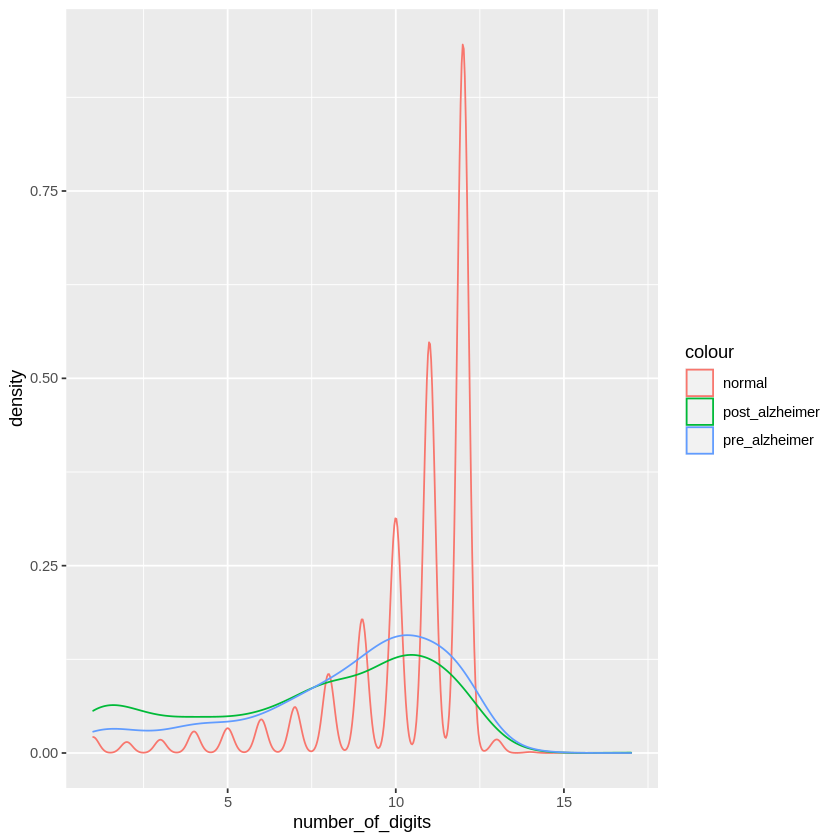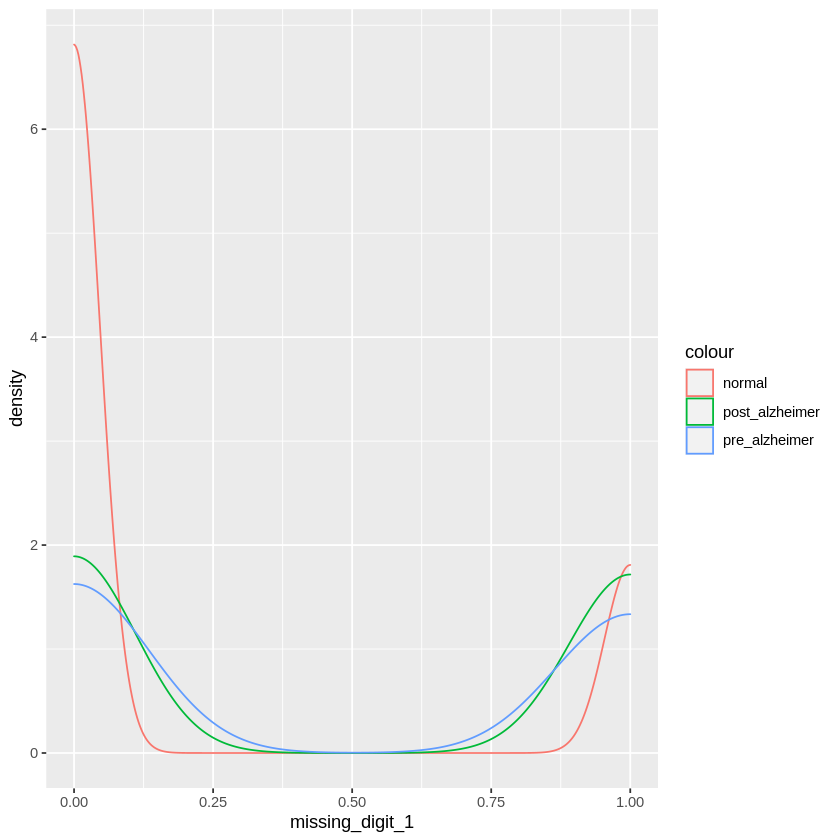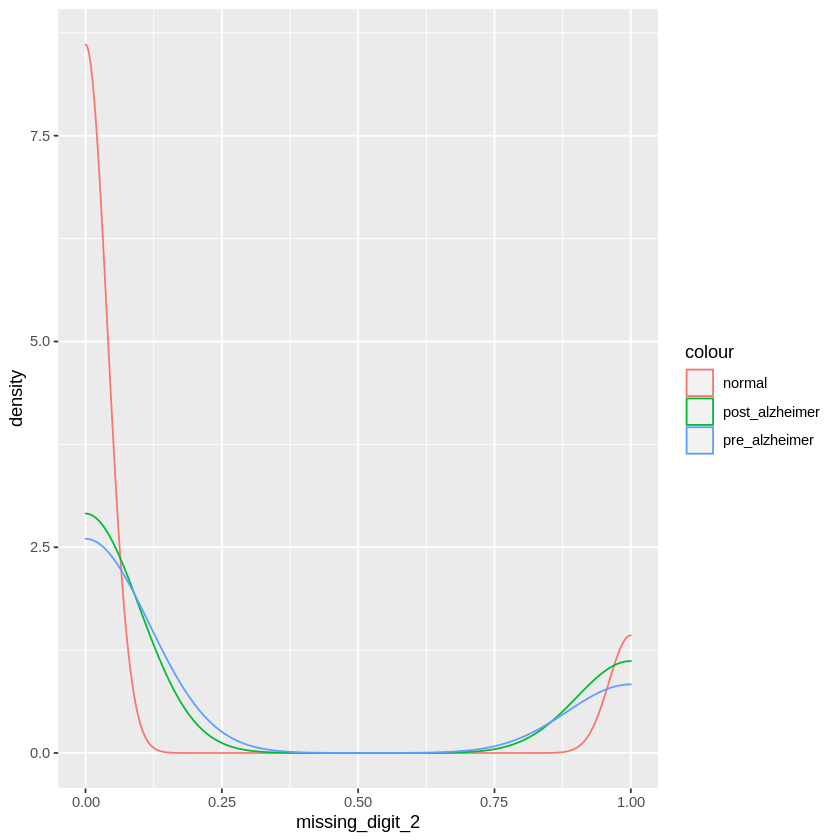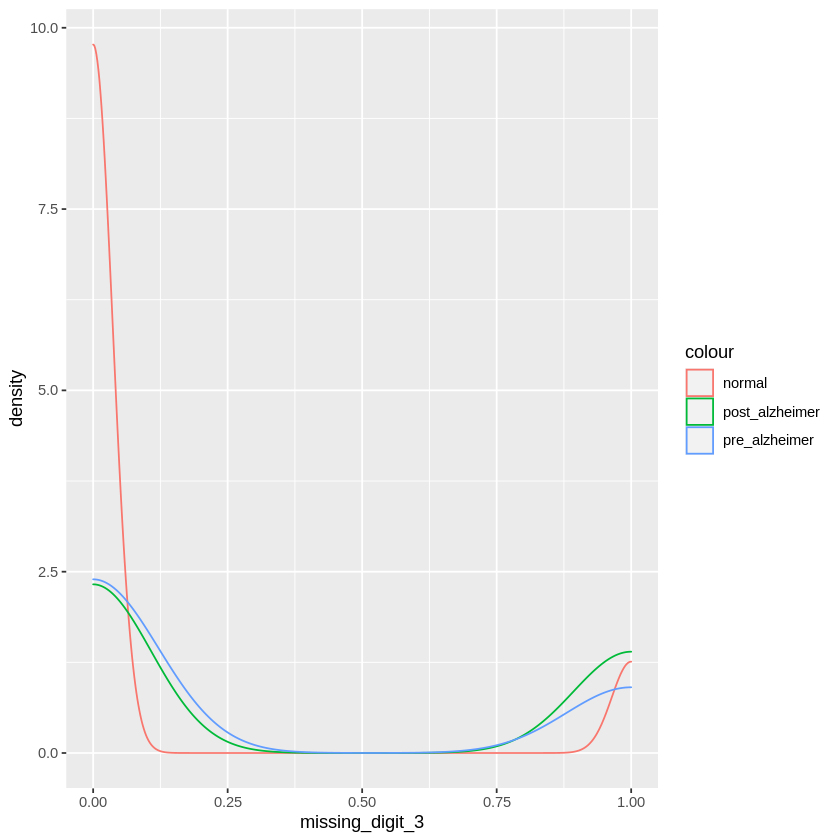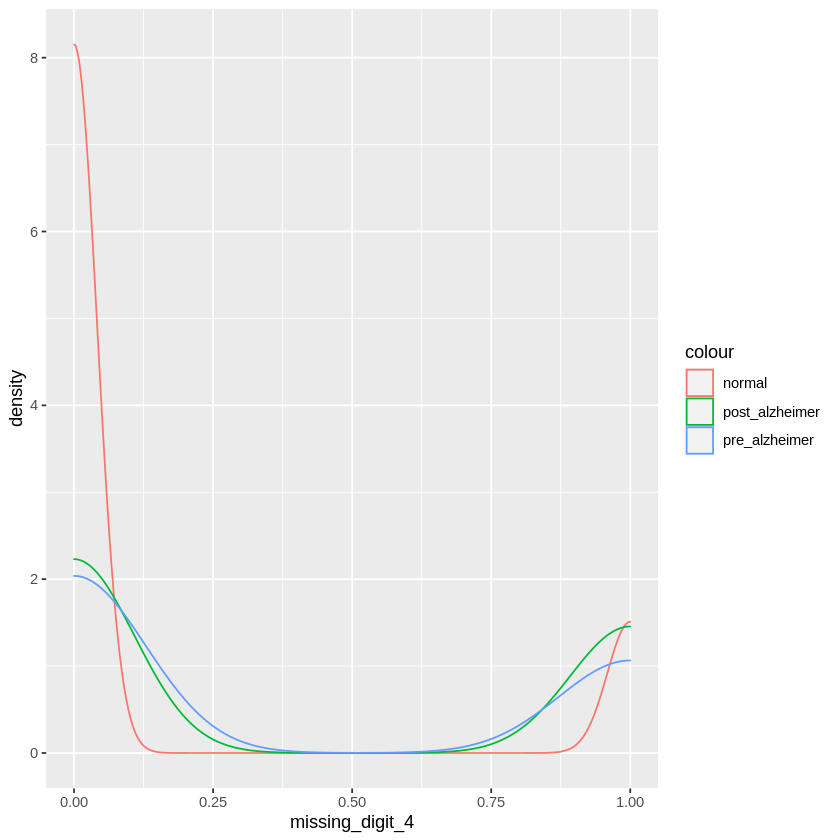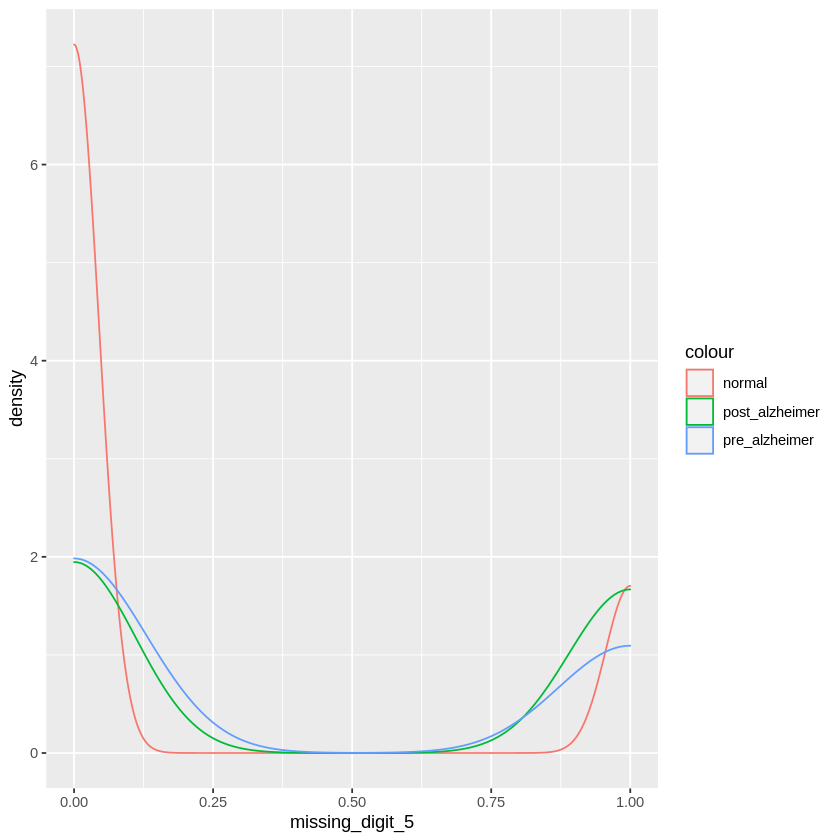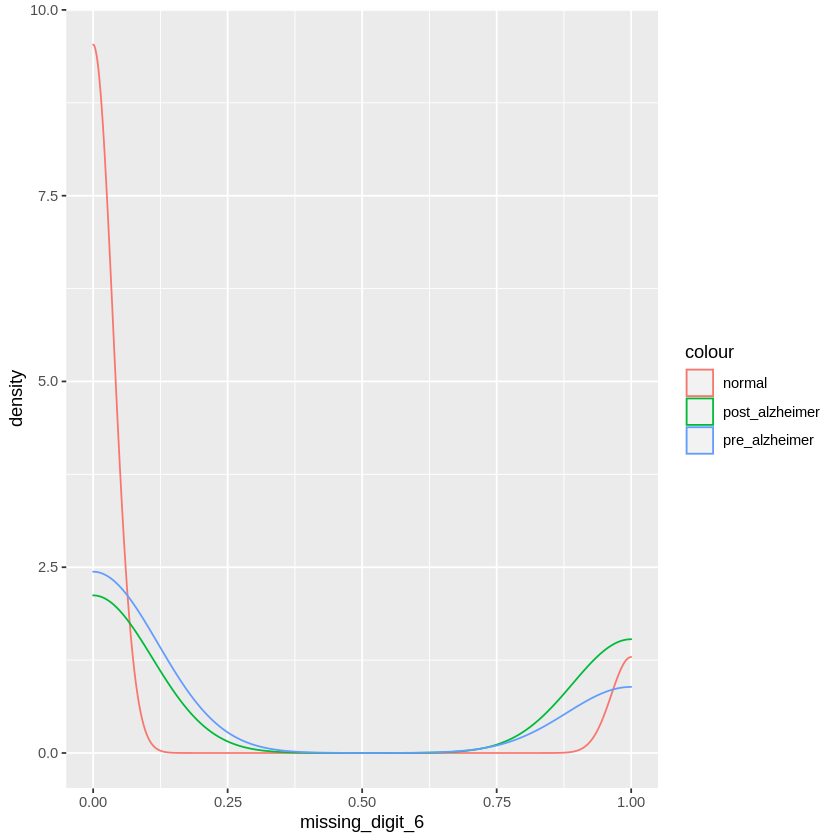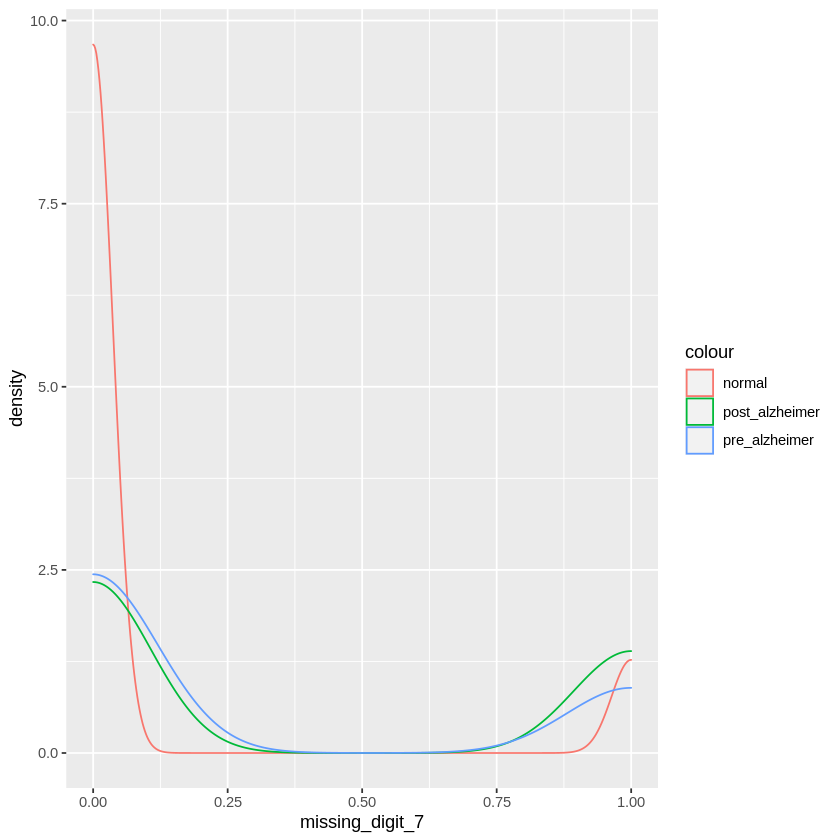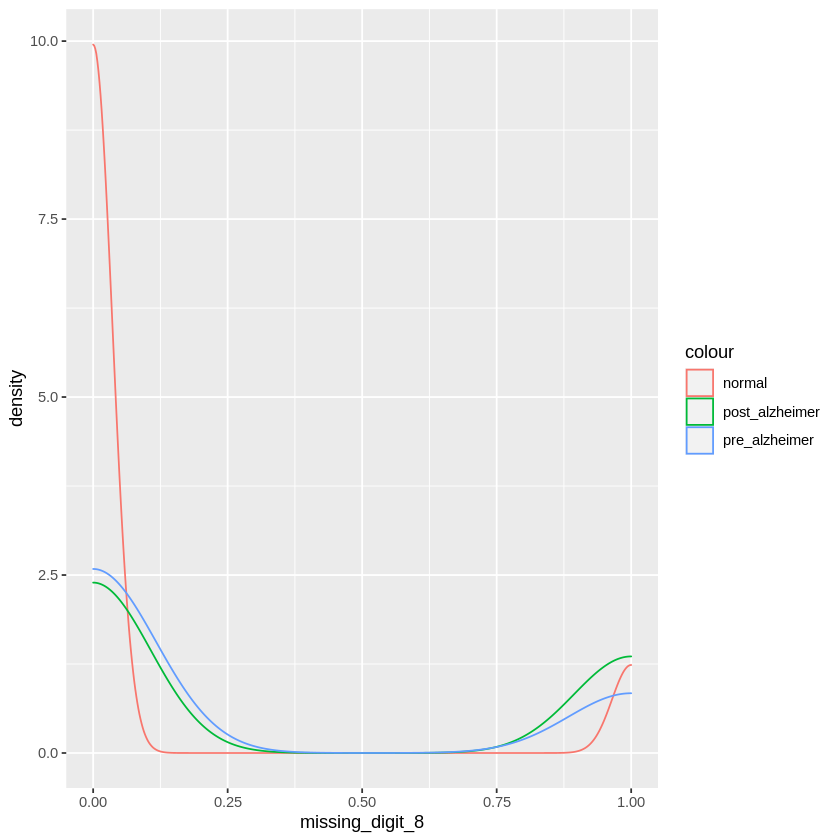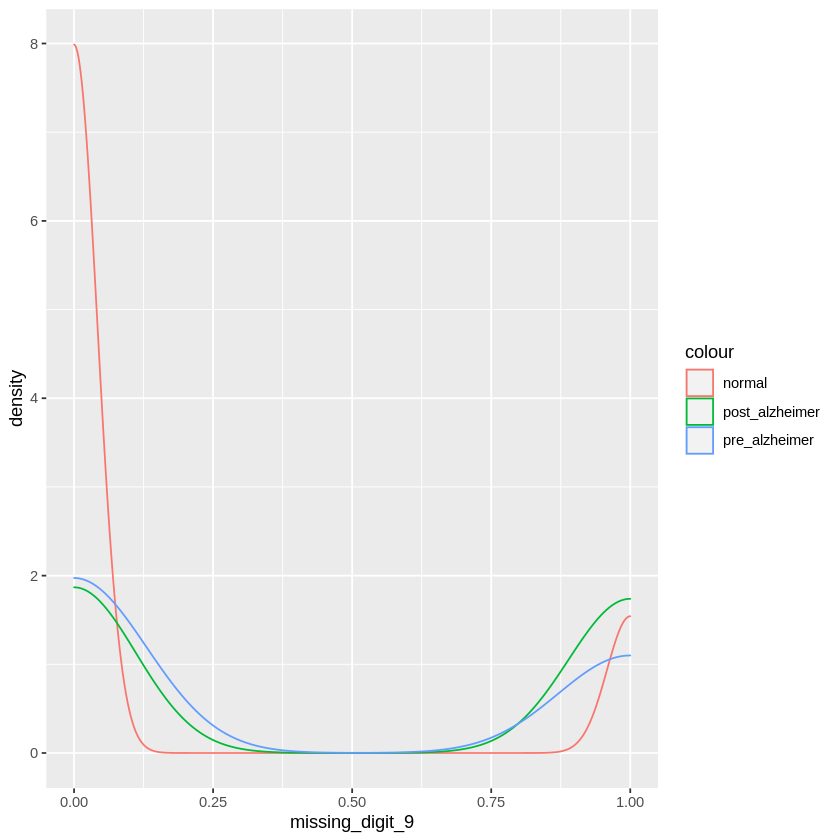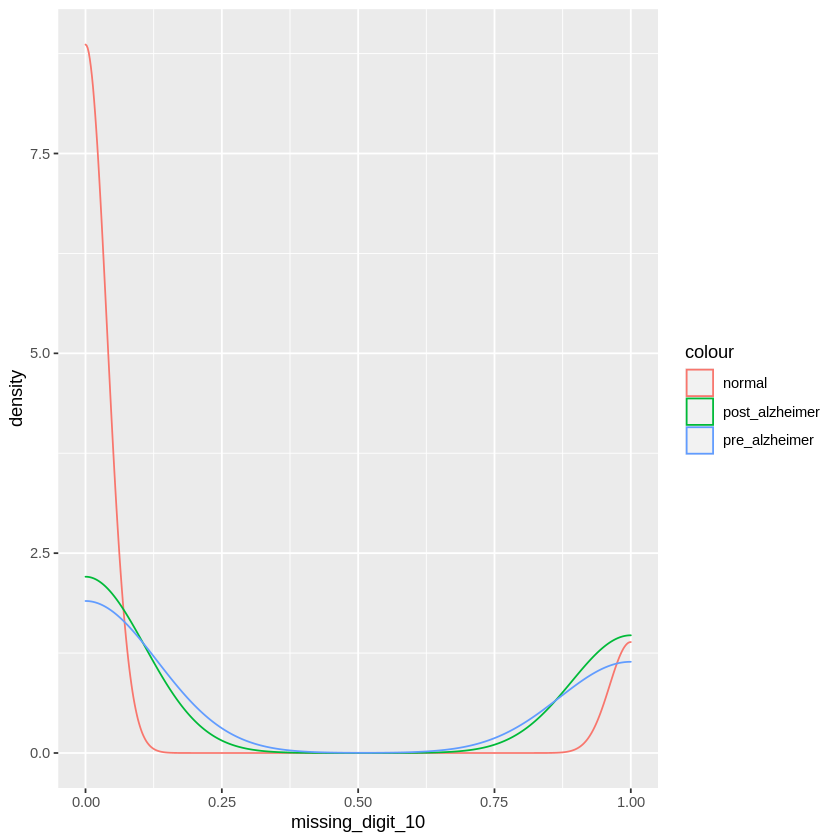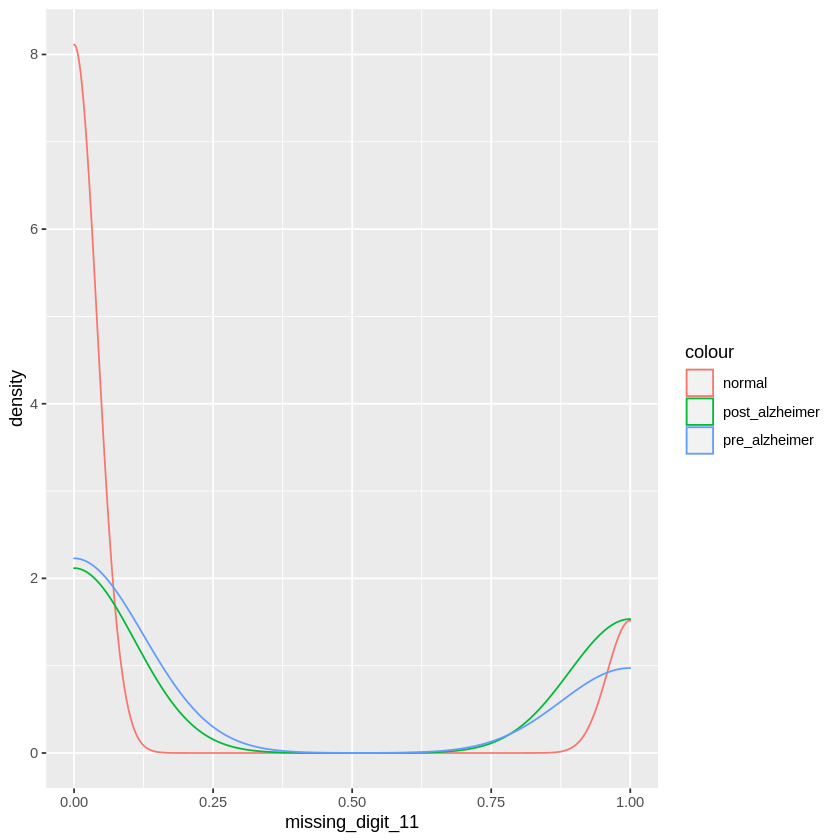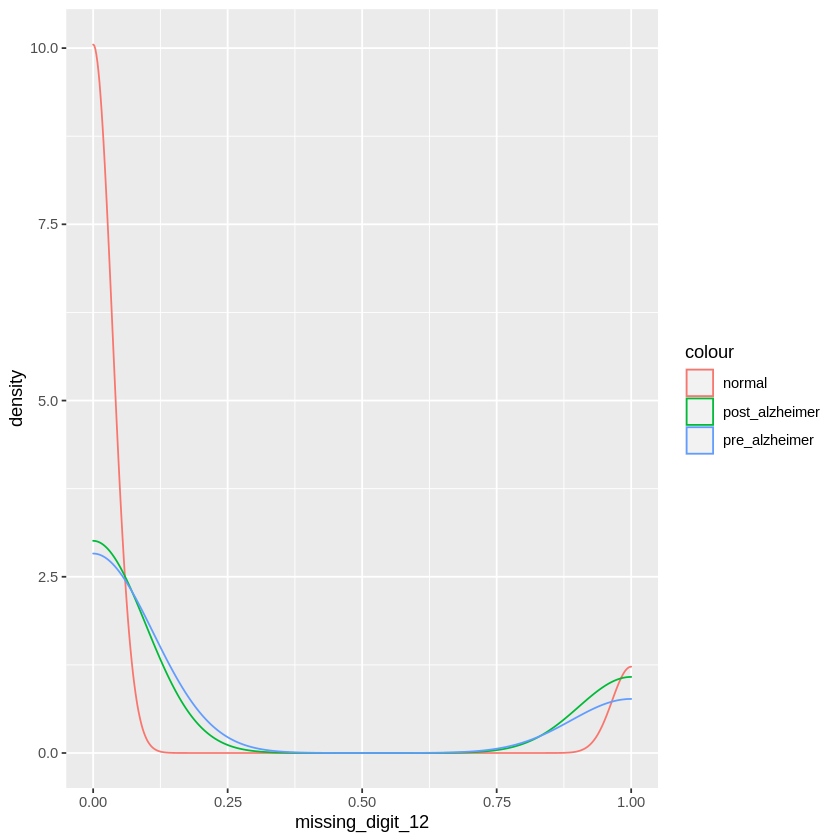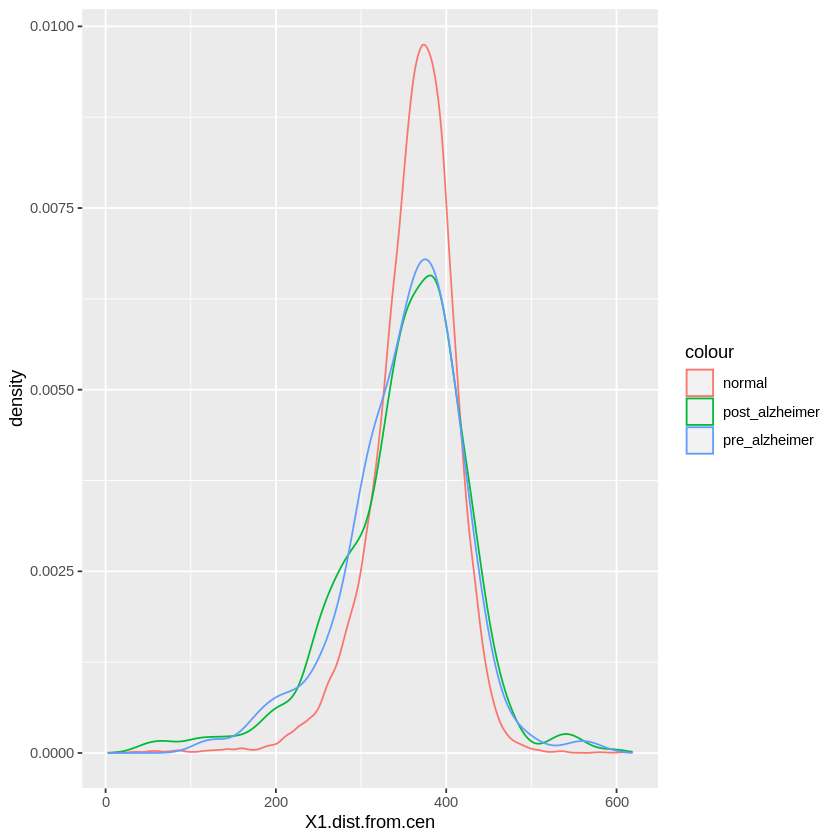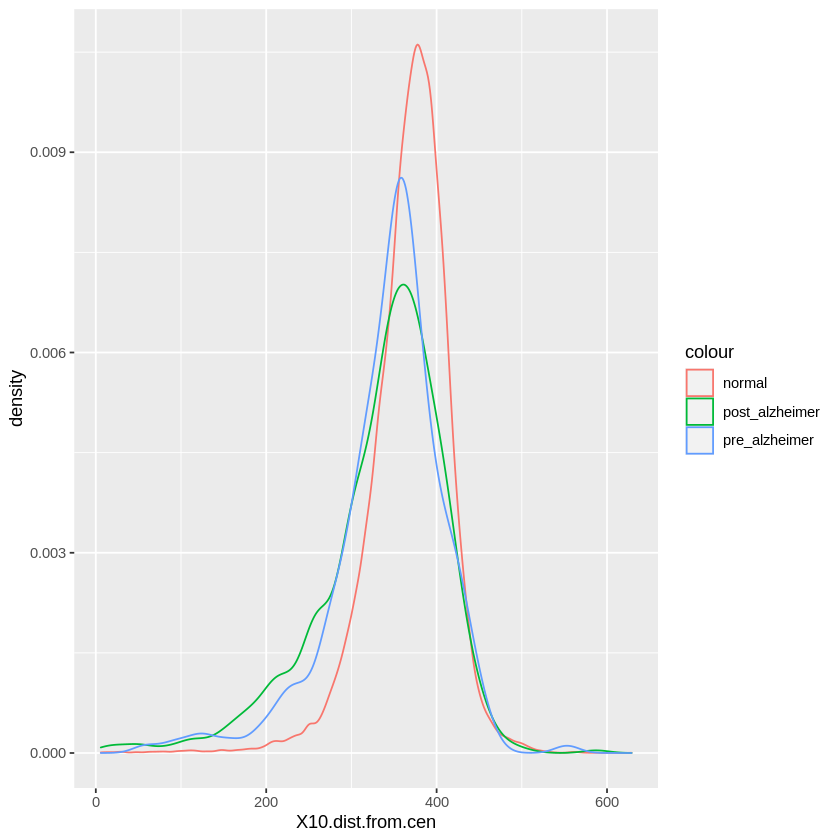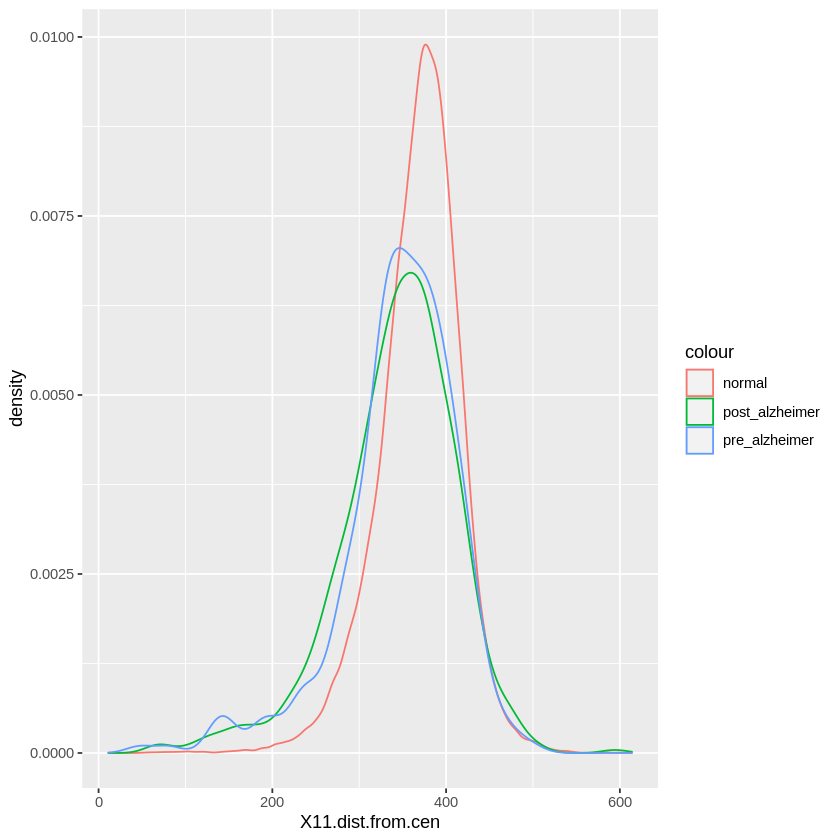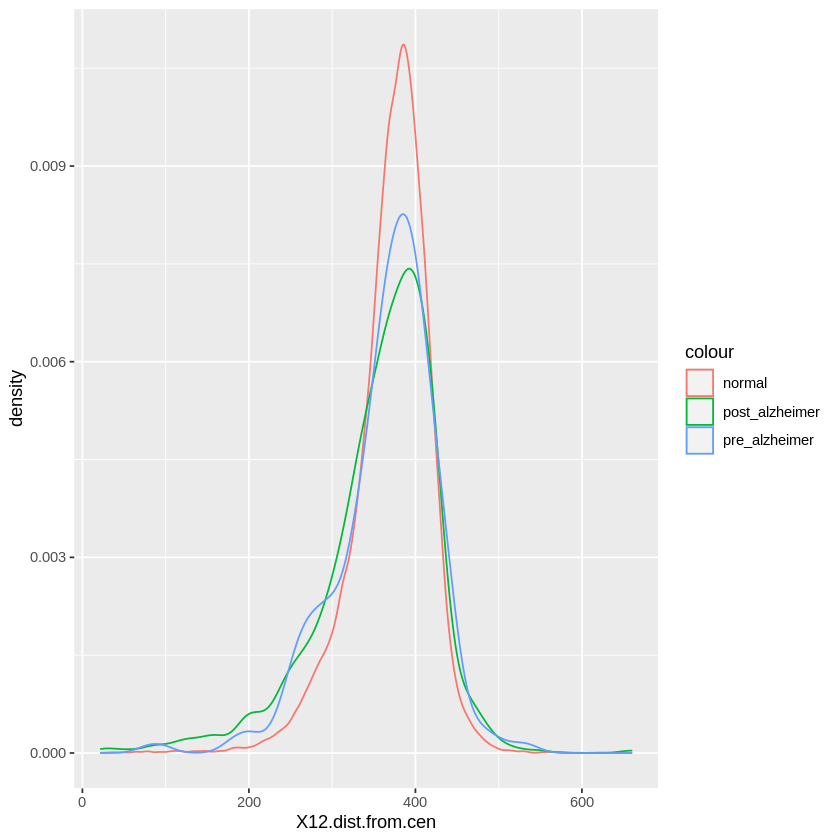# A starter kit for R! +Analysis, Features, Training xgboost

A end-to-end working notebook for R, with light Exploratory Analysis and Feature Engineering

## Hello R user!

Getting up and ready for this ADDI Alzheimer Detection Challenge can be quite challenging. 😰

To help you get started 🏁, here's a starter kit for R,  100% bug-free ! 😁
This was produced on a Linux VM.

Below is the workflow that works, along with:

• Exploratory Analysis 🔍📊
• Feature Engineering 🛠
• Model Training 💻
• Prediction 🔮
• Submission 🚀

This Notebook scored 0.79 on the public leaderboard.
Pro-tip: Rebalancing weights can improve its score.

Enjoy!

Michaël# What is the notebook about?¶

The challenge is to use the features extracted from the Clock Drawing Test to build an automated and algorithm to predict whether each participant is one of three phases:

1) Pre-Alzheimer’s (Early Warning) 2) Post-Alzheimer’s (Detection) 3) Normal (Not an Alzheimer’s patient)

In machine learning terms: this is a 3-class classification task.

# How to use this notebook 📝¶• Update the config parameters. You can define the common variables here
Variable Description
AICROWD_DATASET_PATH Path to the file containing test data (The data will be available at /ds_shared_drive/ on aridhia workspace)
AICROWD_PREDICTIONS_PATH Path to write the output to. This should be an absolute path.
AICROWD_ASSETS_DIR In case your notebook needs additional files (like model weights, etc.,), you can add them to a directory and specify the path to the directory here (please specify relative path). The contents of this directory will be sent to AIcrowd for evaluation.
AICROWD_API_KEY In order to submit your code to AIcrowd, you need to provide your account's API key. This key is available at https://www.aicrowd.com/participants/me
• Installing packages. Please use the Install packages 🗃 section to install the packages
• Training your models. All the code within the Training phase ⚙️ section will be skipped during evaluation. Please make sure to save your model weights in the assets directory and load them in the predictions phase section

# Setup AIcrowd Utilities 🛠¶

We use this to bundle the files for submission and create a submission on AIcrowd. Do not edit this block. These are the dependencies needed for making AIcrowd submission, you can skip this block untouched.

In :
cat(system('curl -sL https://gitlab.aicrowd.com/snippets/34123/raw > aicrowd.R', intern=TRUE), sep='\n')
cat(system('pip3 install -q -U aicrowd-cli', intern=TRUE), sep='\n')
cat(system('pip install -q -U aicrowd-cli', intern=TRUE), sep='\n')




In :
source("aicrowd.R")


# Evaluation configuration 🧷¶

During the evaluation, these variables will get values from environment variables.

You can get your API key from https://www.aicrowd.com/participants/me

In :
# You can get your API key from https://www.aicrowd.com/participants/me

# Please use the absolute for the location of the dataset.
AICROWD_DATASET_PATH = Sys.getenv("DATASET_PATH", "/ds_shared_drive/validation.csv")
AICROWD_PREDICTIONS_PATH = Sys.getenv("PREDICTIONS_PATH", "predictions.csv")

# Use this directory to store any assets needed
AICROWD_ASSETS_DIR = "assets"


# Install packages 🗃¶

Your packages and dependencies listed below will be automatically installed during evaluations.

In :
#install.packages("ggplot2", repos='http://cran.ma.imperial.ac.uk')
#install.packages("dplyr", repos='http://cran.ma.imperial.ac.uk')
#install.packages("stringr", repos='http://cran.ma.imperial.ac.uk')
cat(system('conda install --quiet --yes -c conda-forge r-xgboost', intern=TRUE), sep='\n')

Collecting package metadata (current_repodata.json): ...working... done
Solving environment: ...working... done

# All requested packages already installed.



# Define preprocessing code 💻¶

In :
library(ggplot2)
library(dplyr)
library(stringr)
library(xgboost)

In :
##########################################################################################################
#
# $$\$$\ \ $$\$$\       $$\ #$$ |  $$|$$  _____|$$|$$ |     $$__$$\
# $$|$$ |$$|$$ |      $$|$$ /  $$| #$$$$|$$$$\$$ |      $$|$$ |  $$| #$$  __$$|$$  __|   $$|$$ |     $$|$$ |
# $$|$$ |$$|$$ |      $$|$$ |  $$| #$$ |  $$|$$$$\$$$$\$$$$\$$  |
# \__|  \__|\________|\________|\________|\______/
#
#
# Hello R user!
# I wanted to share a starter kit for R users...  are there any in the competition? :)
#
# Below you will find:
#
#   - light Exploratory Data Analysis
#   - small Feature Engineering
#   - Model Training
#   - Prediction Generating
#   - Working Submission (Leaderboard Log Loss of )
#
#
# I hope this can inspire you, and more importantly, help you debug any issues you might encounter!
#
# Don't hesitate to comment below.
#
# Stay safe, enjoy!
#
# Michaël
#
##########################################################################################################

In :
# Looking at the data, there is quite a few NAs.
#
# However, it appears most of the time that whenever there's a NA, it looks like 0 could be a good proxy.
#
# As an example, for the length of the clock hand.
#  - If the clock hand is absent, it is identified as NA.
#  - But we could reasonably also call it a length of 0.
#
# Therefore, in order to produce the correlation chart, I will replace all NAs with 0.

In :
# names(td)[sapply(td, anyNA)]  #to see variables with NAs

In :
preprocess <- function(df){

# Remove the ID variable
df <- df[, !names(df) %in% c("row_id")]

#Converting character variables to factors
df$intersection_pos_rel_centre <- as.factor(df$intersection_pos_rel_centre)

# Feature Engineering
df$count_NA <- rowSums( is.na( df[,] )) # count the number of NA #Cleaning df[is.na(df)] <- 0 # imputing 0 to missing # Feature Enineering df$ellipse_area = 3.1451926 * df$double_major * df$double_minor / 2    # surface area of the clock

return(df)
}


# Training phase ⚙️¶

You can define your training code here. This sections will be skipped during evaluation.

In :
td = read.csv(str_replace(AICROWD_DATASET_PATH,"validation","train"))

td$diagnosis <- as.factor(td$diagnosis)

In :
# The target variable is a multi-class, with 3 levels.
#
#    normal
#    pre_alzheimer
#    post_alzheimer
#
# We will look at how behaves each feature variable in the context of the three classes to predict.

In :
# Remove the ID variable
td <- td[, !names(td) %in% c("row_id")]

# Number of graphs to produce, and their name
nvar = ncol(td)
#nvar = 5
var_list = combn(names(td)[1:nvar], 1, simplify=FALSE)

# Make plots.
for (i in 1:nvar) {
p = ggplot(td, aes_string(x=var_list[[i]], color=td\$diagnosis)) +
geom_density(alpha=0, na.rm=TRUE)
print(p)
}